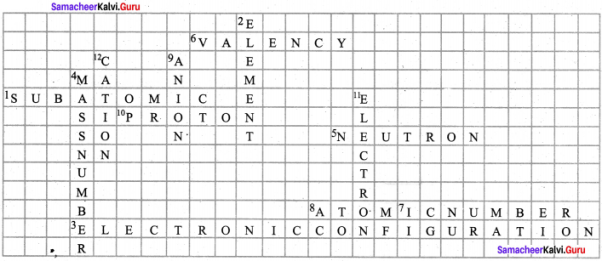## Tamilnadu Samacheer Kalvi 8th Science Solutions Term 2 Chapter 4 Atomic Structure

### Samacheer Kalvi 8th Science Atomic Structure Text Book Exercises

8th Science Atomic Structure Question 1.
The same proportion of carbon and oxygen in the carbon dioxide obtained from different sources proves the law of
(a) reciprocal proportion
(b) definite proportion
(c) multiple proportion
(d) conservation of mass
(b) definite proportion

Atomic Structure Book Back Answers Question 2.
Cathode rays are made up of –
(a) neutral particles
(b) positively charged particles
(c) negatively charged particles
(d) None of the above
(c) negatively charged particles

Atomic Structure Class 9 Samacheer Kalvi Question 3.
In water, hydrogen and oxygen are combined in the ratio of by mass.
(a) 1 : 8
(b) 8 : 1
(c) 2 : 3
(d) 1 : 3
(a) 1 : 8

8th Standard Question 4.
Which of the following statements made by Dalton has not undergone any change?
(a) Atoms cannot be broken.
(b) Atoms combine in small, whole numbers to form compounds.
(c) Elements are made up of atoms.
(d) All atoms of an elements are alike
(d) All atoms of an elements are alike

Question 5.
In all atoms of an element –
(a) the atomic and the mass number are same.
(b) the mass number is same and the atomic number is different.
(c) the atomic number is same and the mass number is different
(d) both atomic and mass numbers may vary.
(a) the atomic and the mass number are same.

II. Fill in the blanks

1. …………….. is the smallest particle of an element.
2. An element is composed of …………….. atoms.
3. An atom is made up of …………….., …………….. and ……………..
4. A negatively charged ion is called …………….. while positively charged ion is called
5. …………….. is a negatively charged particle (Electron/Proton).
6. Proton is deflected towards the …………….. charged plate (positively, negatively).

1. Atom
2. same kind of
3. proton, electron, neutron
4. anion, cation
5. Electron
6. negatively

III. Match the following

1. Law of Conservation of Mass – Sir William Crookes
2. Law of Constant Proportion – James Chadwick
3. Cathode rays – Joseph Proust
4. Anode rays – Lavoisier
5. Neutrons – Goldstein

1. Law of Conservation of Mass – Lavoisier
2. Law of Constant Proportion – Joseph Proust
3. Cathode rays – Sir William Crookes
4. Anode rays – Goldstein

Question 1.
State the Law of Conservation of Mass.
The law states that during any chemical change, the total mass of the products is equal to the total mass of the reactants.

Question 2.
State the Law of Constant Proportions.
Law of constant proportions states that in a pure chemical compound the elements are always present in definite proportions by mass.

Question 3.
Write the properties of anode rays.
Properties of Anode rays:

1. Anode rays travel in straight lines.
2. Anode rays are made up of material particles.
3. Anode rays are deflected by electric and magnetic fields. Since, they are deflected towards the negatively charged plate, they consist of positively charged particles.

Question 4.
Define valency of an element with respect to hydrogen.
Valency of an element is defined as the number of hydrogen atoms which combine with one atom of it.

Question 5.
Define the term ions or radicals.
An atom or a group of atoms when they either lose or gain electrons, get converted into ions or radicals.

Question 6.
What is a chemical equation?
A chemical equation is a short hand representation of a chemical reaction with the help of chemical symbols and formula.

Question 7.
Write the names of the following compounds.

1. CO
2. N2O
3. NO2
4. PCl5

1. Carbon monoxide.
2. Nitrous oxide
3. Nitrogen dioxide
4. Phosphorous pentachloride

Question 1.
Find the valency of the element which is underlined in the following formula.

1. NaCl
2. CO2
3. Al(PO2)
4. Ba(NO3)2
5. CaCl2

1. NaCl = 1
2. CO2 = 4
3. Al(PO4) = 3
4. Ba(NO3)2 = 2
5. CaCl2 = 2

Question 2.
Write the chemical formula for the following compounds

1. Aluminium sulphate
2. Silver nitrate
3. Magnesium oxide
4. Barium chloride

1. Aluminium sulphate = Al2(SO4)3
2. Silver nitrate = AgNO3
3. Magnesium oxide = MgO
4. Barium chloride = BaCl2

Question 3.
Write the skeleton equation for the following word equation and then balance them.

1. Carbon + Oxygen → Carbon dioxide
2. Phosphorus + Chlorine → Phosphorus pentachloride.
3. Sulphur + Oxygen → Sulphur dioxide
4. Magnesium + hydrogen chloride → Magnesium chloride + Hydrogen

Balanced equation:

1. C + O2 → CO2
2. P4 + 10 Cl2 → 4PCl5
3. S + O2 → SO2
4. Mg + 2HCl → MgCl2 + H2

Skeleton equation:

1. C + O2 → CO2
2. P + Cl2 → PCl5
3. S + O2 → SO2
4. Mg + 2HCl → MgCl2 + H2

Question 4.
Balance the following chemical equation.

1. Na + O2 → Na2O
2. Ca + N2 → Ca3N2
3. N2 + H2 → NH3
4. CaCO3 + HCl → CaCl2 + CO2 + H2O
5. Pb(NO3)2 → PbO + NO2 + O2

1. 4Na + O2 → 2Na2O
2. 3Ca + N2 → Ca3N2
3. N2 + 3H2 → 2NH3
4. CaCO2 + 2HCl → CaCl2 + H2O + CO2
5. 2Pb (NO3)2 → 2PbO + 4NO2 + O2

VI. Higher Order Thinking Questions

Question 1.
Why does a light paddle wheel placed in the path of cathode rays begin to rotate, when cathode rays fall on it?
It is because the small particles of the cathode rays (electrons) have mass and energy. This energy is used in rotating the paddle wheels.

Question 2.
How can we prove that the electrons carry negative charge?
J.J. Thomson found that cathode rays were attracted by the positively charged plate and repelled by the negatively charged plate. This led him to the conclusion that the cathode rays (electrons) were made of negatively charged particles.

Question 3.
Ruthresh, Hari, Kanishka and Thahera collected different samples of water from a well, a pond, a river and underground water. All these samples were sent to a testing laboratory. The test result showed the ratio of hydrogen to oxygen as 1:8.

1. What conclusion would you draw from the above experiment?
2. Which law of chemical combination does it obey?

1. Water obtained from different sources like a well, a pond, a river and underground water will always consist of the same two elements hydrogen and oxygen in the ratio 1 : 8 by mass.
2. It obeys the law of constant proportion.

### Samacheer Kalvi 8th Science Atomic Structure Intext Activitics

Activity – 2

Question 1.
Classify the following ions into monovalent, divalent and trivalent.
Ni2+, Fe3+, Cu2+, Ba2+, Cs+, Zn2+, Cd2+, Hg2+, Pb2+, Mn2+, Fe2+, CO2+, Sr2+, Cr3+, Li+, Ca2+, Al3+

• Monovalent ions – Li+, Cs+
• Divalent ions – Ni2+, Cu2+, Ba2+, Zn2+, Cd2+, Hg2+, Pb2+, Mn2+, Fe2+, CO2+, Ca2+, Sr2+
• Trivalent ions – Fe3+, Cr3+, Al3+

Activity – 3

Question 1.
Write the chemical formula of the compounds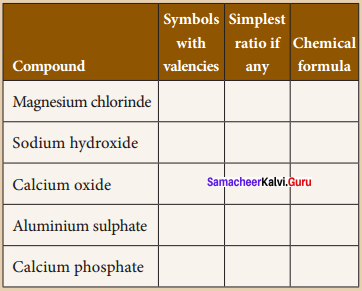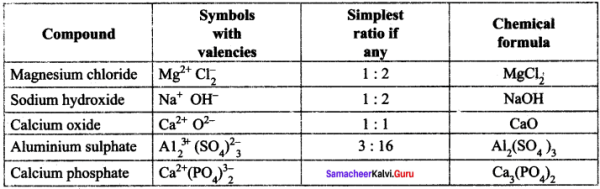Activity – 4

Question 1.
Write the names of the chemical compounds.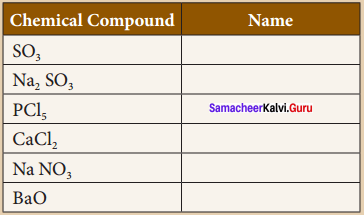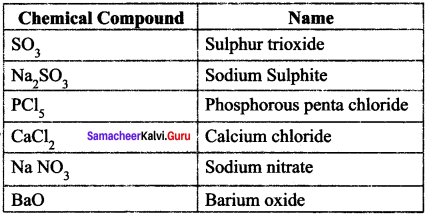### Samacheer Kalvi 8th Science Atomic Structure Additional Questions

I. Choose the correct answer :

Question 1.
Which of the following scientists observed that cathode rays consists of negatively charged particles?
(a) John Dalton
(b) J.J. Thomson
(d) Democritus
(b) J.J. Thomson

Question 2.
The outer most shell of an atom is known as ………………..
(a) valency
(b) valence electron
(c) nucleus
(d) valance shell
(d) valance shell

Question 3.
The valency of which of the element is zero.
(a) iron
(b) hydrogen
(c) helium
(d) oxygen
(c) helium

Question 4.
The equation Na + H2O → NaOH + H2 is ………………
(a) correct
(b) iricorrect since it is not balanced
(c) incorrect since hydrogen should be written as H and not H2
(d) None
(b) ipcorrect since it is not balanced

Question 5.
Whatis the valency of carbon?
(a) 1
(b) 2
(c) 3
(d) 4
(d) 4

Question 6.
Metals form ……………..
(a) anions
(b) cations
(c) both a and b
(d) none
(b) cations

Question 7.
Isotopes exist because atoms of the same element can have different numbers of –
(a) protons
(b) neutrons
(c) electrons
(d) none
(b) neutrons

Question 8.
An atom differs from its ion in ………………
(a) nuclear charge
(b) mass number
(c) number of electrons
(d) number of protons
(c) number of electrons

Question 9.
What is the atomic number of an element whose electronic configuration is 2, 8, 1?
(a) 10
(b) 23
(c) 11
(d) 8
(c) 11

II. Fill in the Blanks

1. Isotopes have the same ……………… number but different ……………… numbers.
2. ……………… have the same mass number but different atomic numbers.
3. Crookes Rays are also called as ……………….
4. Cathode rays are made up of material particles which have mass and ……………….
5. A proton can be defined as a ………………
6. Atoms of all metals will have …………….. electrons in their outermost orbit.
7. All non – metals will have ……………… electrons in the outermost orbit of their atoms.
8. Atoms which carry positive or negative charges are called ……………….
9. Maximum number of electrons that can be present in N shell is ……………….
10. The atomic theory was first proposed by ……………….

1. atomic, mass
2. Isobars
3. Cathode rays
4. kinetic energy
5. hydrogen ion
6. 1 to 3
7. 4 to 7
8. ions
9. 32
10. Dalton

III. True or False – if false give the correct statement

Question 1.
Anode rays travel in straight lines.
True

Question 2.
James Chadwick discovered the fundamental particle called proton.
False
Correct statement:
James Chadwick discovered the fundamental particle called neutron.

Question 3.
Relative charge of an electron is – 1.
True

Question 4.
Atoms of the same element are identical in all respects.
True

Question 5.
The first scientific theory about atom was given by J J. Thomson.
False
Correct statement:
The first scientific theory about atom was given by John Dalton.

Question 6.
In television tube, Cathode rays are deflected by magnetic fields.
True

Question 7.
An atom has a number of orbits and each orbit has protons.
False
Correct statement:
An atom has a number of orbits and each orbit has electrons.

Question 8.
The combining capacity of an atom is called valency.
True

Question 9.
Helium has two electrons in the outermost orbit and so it is chemically inert.
True

Question 10.
When an atom gains an electron it has more number of electrons and thus it carries positive charge.
False
Correct statement:
When an atom gains an electron it has more number of electrons and thus it carries negative charge.

IV. Match the following

Question 1.

 1 J.J. Thomson (a) Law of definite proportions 2 Air (b) Law of indestructibility of mass 3 Hydrogen ion (c) Poor conductor of electricity 4 Law of constant proportions (d) Plum pudding model 5 Law of conservation of mass (e) H+

1. d
2. c
3. e
4. a
5. b

Question 2.

 1 Crooke’s tube (a) Negatively charged particles 2 Cathode rays (b) Cathode ray tube 3 Proton (c) Positively charged particles 4 Anode rays (d) 1.6 x 10-24 g

1. b
2. a
3. d
4. c

V. Assertion and Reason.

Question 1.
Mark the correct choice as :
(a) If both assertion and reason are true and the reason is the correct explanation of the assertion.
(b) If both assertion and reason are true, but the reason is not the correct explanation of the assertion.
(c) If the assertion is true, but the reason is false.
(d) If the assertion is false, but the reason is true.

Question 1.
Assertion : Proton can be defined as a hydrogen ion (H+).
Reason : These protons are produced when one electron is removed from one hydrogen atom.
(a) Both assertion and reason are true and the reason is the correct explanation of the assertion

Question 2.
Assertion : Helium has four electrons in the outermost orbit.
Reason : Neon has eight electron in the outermost orbit.
(d) The assertion is false, but the reason is true

Question 1.
What is an atom?
Atom is the smallest particle of matter that takes part in a chemical reaction.

Question 2.
What is electrical discharge?
When electricity passes through air, remove the electrons*from the gaseous atoms and produces ions. This is called electrical discharge.

Question 3.
What is the charge of a neutron?
Neutron is a neutral particle, that is it carries no charge.

Question 4.
Define electronic configuration.
The arrangement of electrons in the orbits is known as electronic configuration.

Question 5.
Define Orbits.
Electrons revolve around the nucleus in a circular fixed paths is called orbits or shells.

Question 6.
Name the subatomic particles of an atom.
Proton, electron and neutron.

Question 7.
Define nucleus.
The nucleus is the central dense core of the atom consisting of neutrons and protons.

Question 8.
What is a reactant in a chemical equation?
Reactant is the substance that take part in a chemical reaction.

Question 9.
What is a product in a chemical equation?
Product is the substance that are formed in a chemical reaction.

Question 10.
What are the two types of valency?
Positive valency and negative valency.

Question 11.
Write any two advantages of Dalton’s atomic theory.

1. Dalton’s theory explains most of the properties of gases and liquids.
2. This explains the law of chemical combination and the law of conservation of mass.

Question 12.
Write any two limitations of Dalton’s atomic theory.

1. Atom is no longer considered as the smallest indivisible particle.
2. Substances made up of same kind of atoms may have different properties.

Question 13.
What are fluorescent materials?
When invisible radiation falls on materials like zinc sulphite, they emit a visible light (or glow). These materials are called fluorescent.

Question 14.
Write the properties of neutrons.

1. Neutron is a neutral particle, that is, it carries no charge.
2. It has mass equal to that of a proton that is 1.6 x 10-24 g.

Question 15.
An atom is electrically neutral. Give reason.
The total number of protons present in an atom is equal to the total number of electrons, thus balancing the total charge of the atom.

Question 16.
What is atomic number?
The total number of protons present in the nucleus of an atom is called its atomic number.

Question 17.
What is mass number?
The total number of protons and neutrons present in the nucleus of an atom is called as mass number.

Question 18.
Mention any two information conveyed by a balanced chemical equation.

1. Balanced chemical equation gives us both qualitative and quantitative information.
2. We can get quantitative information like the number of molecules/atoms of the reactants and products that is taking part in the reaction.

Question 19.
What is a balanced chemical equation?
A balanced chemical equation is one in which the total number of atoms of any element on the reactant side is equal to die total number of atoms of that element on the product side.

Question 20.
Draw a diagram to show the electronic configuration of sodium atom.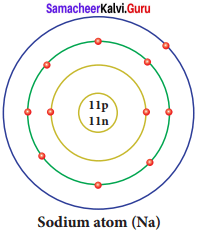Question 21.
Draw a diagram to show the electronic configuration of chlorine atom.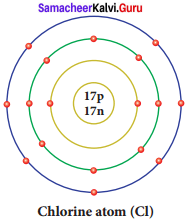Question 22.
Name the four laws of chemical combinations.

1. Law of conservation of mass
2. Law of constant proportion
3. Law of multiple proportion
4. Gay Lussac’s law of gaseous volumes.

Question 23.
Write the names for the following compounds,

1. Aluminium sulphate
2. Silver nitrate.

1. Al2(SO4)3
2. AgNO3

Question 1.

1. Explain J.J. Thomson’s atom model with the help of a diagram.
2. Write the limitations of Thomson’s Atom model.

1. J.J. Thomson’s atom model with the help of a diagram:

• Thomson proposed that the shape of an atom resembles a sphere having a radius of the order of 10-10m.
• The positively charged particles are uniformly distributed with electrons arranged in such a manner that the atom is electrically neutral.
• Thomson’s atom model was also called as the plum pudding model or the watermelon model.
• The embedded electrons resembled the seed of watermelon while the watermelon’s red mass represented the positive charge distribution.
• The plum pudding atomic theory assumed that the mass of an atom is uniformly distributed all over the atom.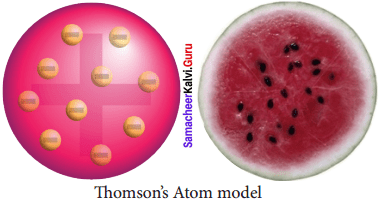2. Limitations of Thomson’s Atom model:

• Thomson’s model failed to explain how the positively charged sphere is shielded from the negatively charged electrons without getting neutralized.
• This theory explains only about the protons and electrons and failed to explain the presence of neutral particle neutron.

Question 2.
Write a note on valency with respect to –

1. Chlorine
2. Oxygen.

1. Valency with respect to Chlorine:

• Since valency of chlorine is one, the number of chlorine atoms with which one atom of an element can combine is called valency.
• In sodium chloride (NaCl) molecule, one chlorine atom combines with one sodium atom. So, the valency of sodium is one.
• But, in magnesium chloride (MgCl2) valency of magnesium is two because it combines with two chlorine atoms.

2. Valency with respect to oxygen:

• Valency can be defined as double the number of oxygen atoms with which one atom of an element can combine because valency of oxygen is two.
• For example, in magnesium oxide (MgO) valency of magnesium is two.

Question 3.
Write the steps are followed to write down the chemical formula of a substance.
Step 1:
Write down the symbols of elements/ions side by side so that the positive radical is on the left and the negative radical is on the right hand side.

Step 2:
Write the valencies of the two radicals above their symbols to the right in superscript (Signs ‘+’ and ‘-‘ of the ions are omitted).

Step 3:
Reduce the valencies to simplest ratio if needed. Otherwise interchange the valencies of the elements/ions. Write these numbers as subscripts. However, ‘1’ appearing on the superscript of the symbol is omitted.

Question 4.
What are the steps involved in writing the skeletal equation?
The following are the steps involved in writing the skeletal equation:

1. Write the symbols and formula of each of the reactants on the left hand side (LHS) and join them by plus (+) sign.
2. Follow them by an arrow (→) which is interpreted as gives or forms.
3. Write on the right hand side (RHS) of arrow the symbols and formula for each of the products.
4. The equation thus written is called as skeleton equation (unbalanced equation).
5. If the product is a gas it should be represented by upward arrow (↑) and if it is a precipitate it should be represented by downward arrow (↓).
Example: Mg + H2SO2 → MgSO4 + H2

Question 5.
What are the points to be followed while balancing a chemical equation?
1. Initially the number of times an element occurs on both sides of the skeleton equation should be counted.

2. An element which occurs least number of times in reactant and product side must be balanced first. Then, elements occuring two times, elements occuring three times and so on in an increasing order must be balanced.

3. When two or more elements occur same number of times, the metallic element is balanced first in preference to non – metallic element. If more than one metal or nonmetal is present then a metal or non-metal with higher atomic mass (refer periodic table to find the atomic mass) is balanced first.

4. The number of molecules of reactants and products are written as coefficient.

5. The formula should not be changed to make the elements equal.

6. Fractional method of balancing must be employed only for molecule of an element (O2, H2,O3, P4,…) not for compound (H2O, NH3,…).

Question 6.
What information are not conveyed by a balanced chemical equation?

1. Physical state of the reactants and the products.
2. Heat changes (heat liberated or heat absorbed) accompanying the chemical reaction.
3. Conditions such as temperature, pressure, catalyst etc., under which the reaction takes place.
4. Concentration (dilute or concentrated) of the reactants and products.
5. Speed of the reaction.

Question 7.
Explain the specific ways to be followed while naming chemical compounds?
1. In naming a compound containing a metal and a non-metal, the name of the metal is written first and the name of the non-metal is written next after adding the suffix-ide to its name.
Examples:
NaCl – Sodium chloride
AgBr – Silver bromide

2. In naming a compound containing a metal, a non-metal and oxygen, name of the metal is written first and name of the non-metal with oxygen is written next after adding the suffix- ate (for more atoms of oxygen) or -ite (for less atoms of oxygen) to its name.
Examples:
Na2 SO4 – Sodium sulphate
Na 2 – Sodium nitrite

3. In naming a compound containing two non – metals only, the prefix mono, di, tri, tetra, penta etc. is written before the name of non – metals.
Examples:
SO2 – Sulphur dioxide
N2O5 – Dinitrogen pentoxide.

Question 8.
Derive the chemical formula for calcium chloride.

• Step 1 – Write the symbols of calcium and chlorine side by side. Ca Cl
• Step 2 – Write the valencies of calcium and chlorine above their symbols to the right. Ca2 Cl1.
• Step 3 – Interchange the valencies of elements. Ca Cl2.

Thus the chemical formula for calcium chloride is CaCl2.

Question 9.
Describe an experiment to prove that during a chemical charge, the total mass of matter remains the same.
1. Prepare 5% of barium chloride (5g of BaCL in 100 ml of water) and sodium sulphatesolutions separately.

2. Take some solution of sodium sulphate in a conical flask and some solution of barium chloride in a test tube.

3. Hang the test tube in the conical flask.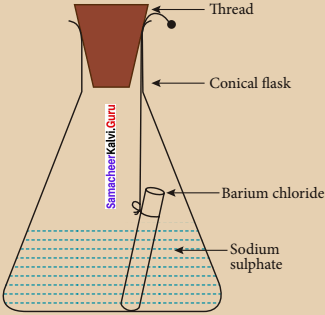4. Weigh the flask with its contents. Now mix the two solutions by tilting and swirling the flask. Weigh the flask after the chemical reaction is occurred.

5. Record your observation. It can be seen that the weight of the flask and the contents remains the same before and after the chemical change.

6. Hence, it is proved that during a chemical change, the total mass of matter remains the same.

Question 10.
Explain the advantages and limitations of Dalton’s atomic theory.

1. Dalton’s theory explains most of the properties of gases and liquids.
2. This explains the laws of chemical combination and the law of conservation of mass.
3. This theory helps to recognize the molecular differences of elements and compounds.

Limitations of Dalton’s Atomic Theory:

1. Atom is no longer considered as the smallest indivisible particle.
2. Atoms of the same element have different masses (Isotopes).
3. Atoms of the different elements may have same masses (Isobars).
4. Substances made up of same kind of atoms may have different properties
5. Example: Coal, Graphite and Diamond are made up of carbon atoms but they differ in their properties

Question 11.
Explain the construction of cathode Ray Tube (CRT) with a diagram.

1. Cathode Ray Tube is a long glass tube filled with gas and sealed at both the ends.
2. It consists of two metal plates (which act as electrodes) connected with high voltage.
3. The electrode which is connected to the negative terminal of the battery is. called the cathode (negative electrode).
4. The electrode connected to the positive terminal is called the anode(positive electrode).
5. There is a side tube which is connected to a pump.
6. The pump is used to lower the pressure inside the discharge tube.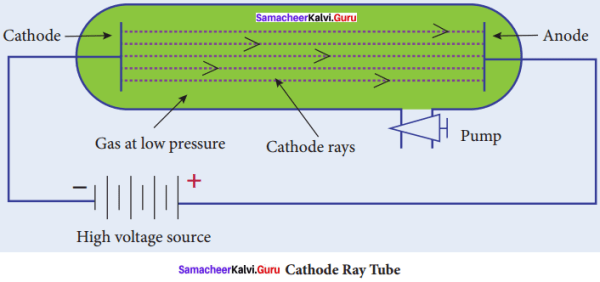Question 12.
Explain the properties of Cathode rays.
Properties of Cathode rays:

1. Cathode rays travel in straight line from cathode towards anode.
2. Cathode rays are made up of material particles which have mass and kinetic energy.
3. Cathode rays are deflected by both electric and magnetic fields. They are negatively charged particles.
4. The nature of the cathode rays does not depend on the nature of the gas filled inside the tube or the cathode used.

Question 13.
Write the main postulates of Dalton’s Atomic theory.
The nain postulates of Dalton’s atomic theory are:

1. All the matters are made up of extremely small particles called atoms (Greek philosopher Democritus used the same name for the smallest indivisible particles).
2. Atoms of the same element are identical in all respects (size, shape, mass and properties).
3. Atoms of different elements have different sizes and masses and possess different properties.
4. Atoms can neither be created nor be destroyed, i.e., atom is indestructible.
5. Atoms of different elements may combine with each other in a fixed simple ratio to form molecules or compounds.
6. An atom is the smallest particle of matter that takes part in a chemical reaction.

Question 14.
Complete the table: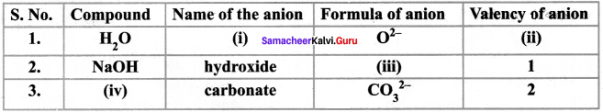1. Oxide
2. 2
3. OH
4. H2CO3

VIII. Solve the following crossword puzzle with the help of the given clues.

Question 1.
Across:
1. The particles that build up an atom.
3. The arrangement of electrons in a succession of energy levels or shells.
5. An electrically neutral sub atomic particle that is part of the nucleus of an atom.
6. A whole number that represents the ability of an atom or a group of atoms to combine with other atoms or group of atoms.
7. The number of protons in the nucleus of an atom.
8. The smallest unit of an element that maintains the properties of an element.
A sub – atomic particle that has a positive electric charge.
Down:
2. A substance that cannot be broken down into simpler substance by chemical means.
4. The total number of protons and neutrons present in the nucleus of an atom.
9. An ion that has a negative charge.
11 A sub – atomic particle with a negative electric charge.
12. Positively charged ions.